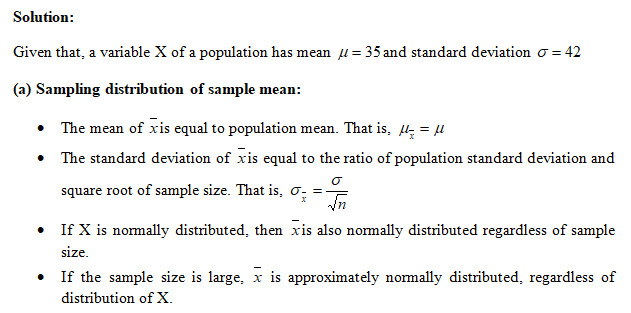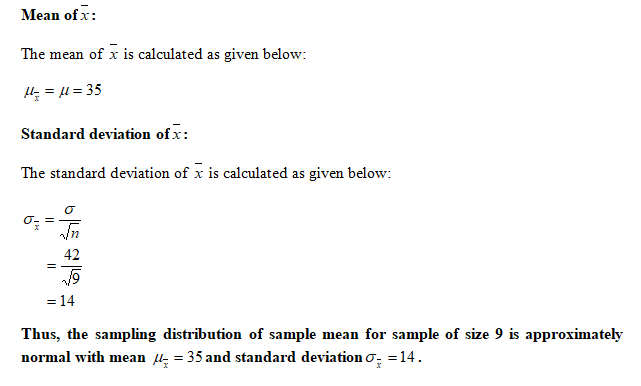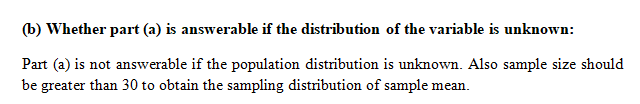# A variable (X) of a population has a mean of 35 and a standard deviation of 42.a. If the variable is normally distributed, describe (shape, center, spread) the sampling distribution of the sample mean for samples of size 9.b. Can you answer part (a) if the distribution of the variable under consideration is unknown? Explain.c. Can you answer part (a) if the distribution of the variable under consideration is unknown, but the sample size is 36 instead of 9? Explain.

Question
2 views

A variable (X) of a population has a mean of 35 and a standard deviation of 42.

a. If the variable is normally distributed, describe (shape, center, spread) the sampling distribution of the sample mean for samples of size 9.

b. Can you answer part (a) if the distribution of the variable under consideration is unknown? Explain.

c. Can you answer part (a) if the distribution of the variable under consideration is unknown, but the sample size is 36 instead of 9? Explain.

check_circle

Step 1Step 2Step 3...

### Want to see the full answer?

See Solution

#### Want to see this answer and more?

Solutions are written by subject experts who are available 24/7. Questions are typically answered within 1 hour.*

See Solution
*Response times may vary by subject and question.
Tagged in